Select Page

Gogus and Boucher (1998) developed a method for calculating the inconsistency ratio of fuzzy pairwise comparison matrices, which its steps are given as follows.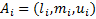STEP 1: Transform a triangular fuzzy matrix into two independent matrices. At this step, a triangular fuzzy matrix is divided into two matrices, assuming that the triangular fuzzy number is presented as follows.   Then, we will get,   The first matrix can be created by middle numbers of the triangular fuzzy matrix, that is: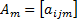The second matrix can be created by the geometric mean (GM) of the upper and lower bounds of the triangular fuzzy matrix, that is: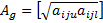STEP 2: Compute the weight vector based on the hourly method and calculation of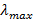STEP 3: Calculate the consistency index (CI): for each matrix, the CI can be calculated based on the following equation: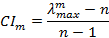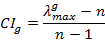STEP 4: Calculate the consistency ratio (CR) of the matrices in Problem. In order to compute the CR, the consistency index (CI) of each matrix is divided by its random index (RI).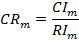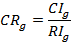If the values of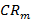andare less than 0.1, the matrices are consistent.   The values of the RI given by Gogus and Boucher method are different from those in the hourly method. Gogus and Boucher redeveloped the random indices (RI) Table for fuzzy pairwise comparison matrices with creating 400 random matrices. The following table presents the values of the RI for each matrix of Gogus and Boucher method.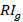n 1 0 1 2 0 2 0.1796 0.4890 3 0.2627 0.7937 4 0.3597 1.0720 5 0.3818 1.1996 6 0.4090 1.2874 7 0.4164 1.3410 8 0.4348 1.3793 9 0.4455 1.4095 10 0.4536 1.4181 11 0.4776 1.4462 12 0.4691 1.4555 13 0.4804 1.4913 14 0.4880 1.4986 15